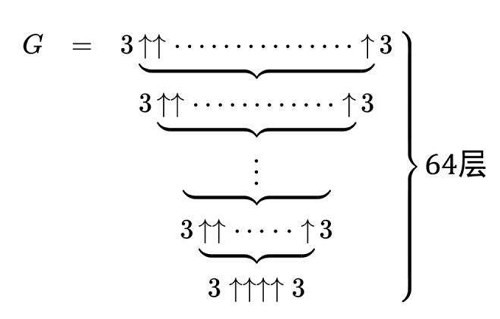# 葛立恒数

## 概述

2018年过年的时候, 在李永乐老师的视频里了解到"葛立恒数"这个东西, 现在呢, 自己想记录一下.

## 基本知识

• 加法是一级运算;
• 乘法是二级运算(加法的快捷方式);
• 乘方是三级运算(乘法的快捷方式);
• 什么是四级运算(乘方的快捷方式)? 下面的高德纳箭头可以说是;

$a \uparrow^{n} b = \begin{cases} 1, &\text{if } b=0 \\ a^{b}, &\text{if } n=1 \\ a \uparrow^{n-1}(a \uparrow^{n}(b - 1)), &\text{其他 } \end{cases}$

### 高德纳箭头运算举例

• $3 \uparrow{2} = 3^{2} = 9$;
• $3 \uparrow{3} = 3^{3} = 27$;
• $3\uparrow \uparrow 3 = 3 \uparrow 3 \uparrow 3 = 3 \uparrow (3 \uparrow 3) = 3 \uparrow 27 = 3^{27} \approx7.6 \times 10^{12}$
• $3 \uparrow \uparrow \uparrow 3 = 3 \uparrow \uparrow (3 \uparrow \uparrow 3)= 3 \uparrow \uparrow 3^{27}=\underbrace{3 \uparrow 3 \uparrow ... \uparrow 3}_{3^{27}个3}=\underbrace{3^{3^{.^{.^{.^{3}}}}}}_{3^{27}个3}$## 参考©️2019 CSDN 皮肤主题: 大白 设计师: CSDN官方博客